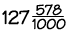Home    |    Teacher    |    Parents    |    Glossary    |    About UsOur decimal system of numbers lets us write numbers as large or as small as we want, using a secret weapon called the decimal point. In our number system, digits can be placed to the left and right of a decimal point, to indicate numbers greater than one or less than one. The decimal point helps us to keep track of where the "ones" place is. It's placed just to the right of the ones place. As we move right from the decimal point, each number place is divided by 10.

We can read the decimal number 127.578 as "one hundred twenty seven and five hundred seventy-eight thousandths". But in daily life, we'd usually read it as "one hundred twenty seven point five seven eight."
Here is another way we could write this number:Notice that the part to the right of the decimal point, five hundred seventy-eight thousandths, can be written as a fraction: 578 over 1000. However, you will hardly ever see a decimal number written like this.
Why do you think this is? You can see that our decimal code is a very handy and quick way to write a number of any size!

Examples

Here's how to write these numbers in decimal form:

Three hundred twenty-one and seven tenths
321.7

(6 x 10) + (3 x 1) + (1 x 1/10) + (5 x 1/100)
63.15

Five hundred forty-eight thousandths
0.548

Five hundred and forty-eight thousandths
500.048

Hint #1: Remember to read the decimal point as "and" -- notice in the last two problems what a difference that makes!

Hint #2: When writing a decimal number that is less than 1, a zero is normally used in the ones place:

0.526 not .526

 Homework Help | Pre-Algebra | NumbersEmail this page to a friendSearch·  Place value·  Decimal numbers·  Estimating and     rounding·  Adding / subtracting     decimals·  Multiplying decimals·  Dividing decimals·  Percent·  Exponents·  Square roots·  Signed integers·  Adding and     subtracting integers·  Multiplying and     dividing integers·  Properties of integersFirst Glance In Depth Examples WorkoutDecimal numbers